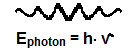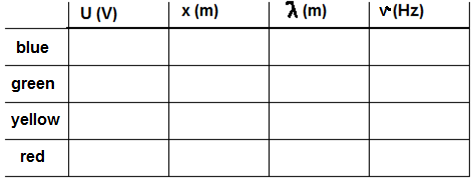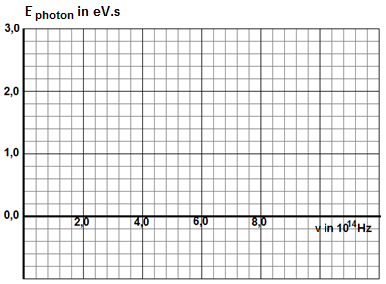### O příspěvku

• SŠ/VŠ

#### Pomůcky

• S jednoduchými pomůckami
• S běžným vybavením kabinetu

## Measuring Planck´s konstant with LED´s

De Jong G.### Background

The relation between the frequency ν of an electromagnetic photon and its energy Ephoton is described by Planck's constant. In the shape of a formula this yields: Ephoton = h×ν.The unit of this constant is joule·second. The energy is calculated in joule. But the unit of Planck's constant can also be eV×s. Now the energy is calculated in eV (1 eV = 1.6×10-19 J approximately).

### The purpose of the experiment

The purpose of this experiment is the measurement of Planck's constant. This is done with monochromatic LED's of various colors.

### Theory

LED's are light sources that emit light of a specific color. The energy of the emitted photon prescribes the voltage that is needed to generate this photon. When a LED emits a photon with the energy of 2.45 eV, then the LED needs a voltage of 2.45 Volt. The voltage over the LED is measured. This voltage directly gives the energy Ephoton of the emitted photon in eV. Next the wavelength of the photon is determined with a diffraction grating slide. The angle α between the light beam from the LED and the first order maximum behind the diffraction grating slide can be calculated with: $$\tan(\alpha) = \frac{x}{l}$$ (see the drawing in the next section). The grating width is: $= \frac{1.0\cdot10^{-3}}{lines\ per\ mm}.$

With these data the wavelength λ of the photon can be calculated: $$\lambda = d \sin(\alpha)$$. This wavelength can be used to calculate the frequency ν: $$\nu = \frac{c}{\lambda}$$. So Planck's constant h is now: $$h = \frac{E_{\mathrm{photon}}}{\nu}$$.

### Set-up

On a base of plywood is a strip with a scale division. Four LED's are mounted in the center: a blue, green, yellow and red one. These LED’s can be activated with a carrousel button behind the strip. The voltage over a single LED can be adjusted with a potentiometer. The voltage is measured with a digital voltmeter. The first order maximum can be spotted through the diffraction grating slide. The position can be determined on the scale division.### Operation procedure

The experiment is carried out in an obscured room, so you will need a small pocket light. Set the multimeter on 20 V DC. Activate the first LED and adjust the voltage in such a way that the LED is just giving off a trace of light. Write down the value of the voltage. Then turn up the voltage. Look through the diffraction grating slide and determine the position of the first order maximum. If the LED is not monochromatic then there will be several maxima. Just take the one closest to the center. Write down the distance of the first order maximum to the center: x. Do this on both sides and calculate the average of both readings. Write the value of x down in the table below. Repeat this procedure for all the other LED's.

### Evaluation

Fill in the first two columns with the values of the voltage and the average distance x.

Calculate the values required in the two remaining columns.

Draw a graph of the voltage U (and thus the photon energy: Ephoton in eV) against the frequency ν. Draw a straight line and determine the slope. This is Planck's constant in eV×s. The unit of the constant can be converted to J×s by multiplying with 1,602×10-19.You can also use a graphic calculator to draw a graph of Ephoton against ν. Chose the option of a straight line. Planck's constant will be the a in "y = a·x + b".### Conclusion

Check the experimental obtained value of Planck's constant with the value that can be found in literature. Determine whether the experiment has been carried out properly and evaluate whether there are any improvements that can be made.